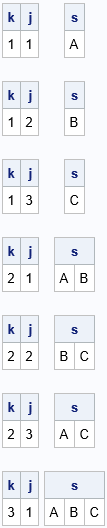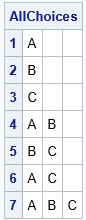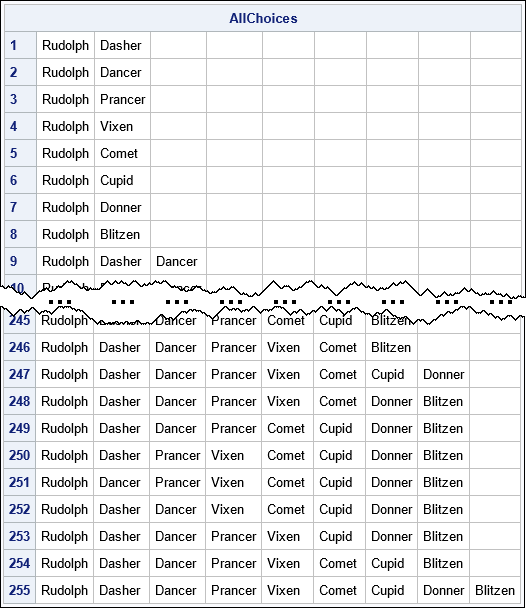A colleague posted a Christmas-themed code snippet that shows how to use the DATA step in SAS to output all the possible ways that Santa can hitch up a team of reindeer to pull his sled. The assumption is that Rudolph must lead the team, and the remaining reindeer are chosen from his loyal team of Dasher, Dancer, Prancer, Vixen, Comet, Cupid, Donner, and Blitzen. The size of the team can be as small as two or as large as nine.

This is a classic mathematical problem: list all the combinations of eight items (the reindeer) taken k at a time. Here k determines the size of the team that pulls the sled. For k=1, the team is Rudolph and one other reindeer. For k=2, the team is Rudolph and two other reindeer, and so on up to k=8.

SAS supports several ways to work with combinations of various sizes. My favorite way is to use the ALLCOMB function in SAS IML software. Santa's reindeer provides a motivation to look at how to use the ALLCOMB function to generate combinations of items of various sizes.

### Generate all combinations

A fundamental rule of programming is to start with an example that is easy to program and understand. So instead of generating all combinations of eight items, let's start with generating all combinations of three items, A, B, and C. The following SAS IML program creates a three-element set. The call to the ALLCOMB function creates all subsets of size k. The call is within a DO loop, so the program creates all subsets of one item, two items, and three items. The result of the ALLCOMB function is k-column matrix where each row is a combination from the set {1, 2, 3}. Each row contains indices that you can use to extract the corresponding subsets from the set {A, B, C}. The following program prints the subsets for each value of k:

```/* 1. All combinations of n elements taken k at a time, k=1:n */ proc iml; set = {"A" "B" "C"}; n = ncol(set);   do k = 1 to n; /* look at subsets of size k */ p = allcomb(n, k); /* each row is a combination "n choose k" */ do j = 1 to nrow(p); /* the j_th subset of size k */ s = set[ , p[j,]]; /* extract the j_th combination (subset) */ print k j, s; end; end; QUIT;```The tricky statement is s = set[ , p[j,]], so let's break that down. The expression p[j,], is the j_th row of the matrix, p. This is a row vector of length k that contains indices. For example, when k=2, the rows are {1 2}, {2 3}, and {1 3}. These indices are used to extract subsets of the set. For example, set[ , {1 3}] is the row vector {A C}. When k=2, the DO loop generates the subsets {A B}, {B C}, and {A C}.

Typically, we do not want to print the output but want to save the output in a matrix or write the output to a data set. The next section puts the results in a matrix. If you want, it is easy to write a SAS IML matrix to a SAS data set.

### Store all combinations as rows in matrix

To store the combinations in a matrix, it is useful to know the total number of combinations. For a set that has n elements, the set of all combinations of size k is given by the binomial coefficient "n choose k," which I will write as comb(n,k). By using the binomial formula from high-school algebra, you can show that the sum of the binomial coefficients is Σk > 0 comb(n,k) = 2n – 1.

The following program is almost identical to the previous one. The difference is that the program allocates a matrix that has n columns and 2n – 1 rows. Instead of printing the subsets, the subsets are assigned to the rows of the matric, as follows:

```/* 2. Store all combinations as rows in a (2##n - 1) x n matrix */ proc iml; set = {"A" "B" "C"}; n = ncol(set); nAllComb = 2##n - 1; /* = sum( comb(n, 1:n) ) */ AllChoices = repeat(BlankStr(nleng(set)), nAllComb, n); /* matrix to store all combinations */   cnt = 1; do k = 1 to n; /* look at subsets of size k */ p = allcomb(n, k); /* each row is a combination "n choose k" */ colIdx = 1:k; /* put combination in column 1:k */ do j = 1 to nrow(p); /* the j_th subset of size k */ s = set[ , p[j,]]; /* extract the j_th combination */ AllChoices[cnt,colIdx] = s; /* put j_th combination into row of a matrix */ cnt = cnt + 1; end; end; print AllChoices[r=(1:nAllComb)]; QUIT;```### Vectorize to improve performance

In a matrix language, you should always be alert for opportunities to improve performance by vectorizing a computation. Often, a clue that you can vectorize is when you notice a loop over all rows (or column) of a matrix. In the previous section, the inner DO loop is a loop over the rows of the matrix, p. That means that every row of p is used to extract elements of the set.

You might be surprised to learn that you can use the matrix p as an index to extract all subsets in one call. The expression set[p] returns a row vector that contains all elements in the set that are indexed by the elements of p. For example, when k=2, the elements of p (in row-major order) are {1 2 2 3 1 3}. Therefore, the expression set[p] resolves to the vector {A B B C A C}. If you reshape these elements into a matrix that has k columns, you get all pairwise combinations of the set {A B C} in a matrix. You an insert these rows into the rows of the matrix that holds the final results, as shown in the following program:

```/* 3. Vectorize: eliminate the loop over j */ proc iml; set = {"A" "B" "C"}; n = ncol(set); nAllComb = 2##n - 1; /* = sum( comb(n, 1:n) ) */ AllChoices = repeat(BlankStr(nleng(set)), nAllComb, n);   cnt = 0; do k = 1 to n; /* look at subsets of size k */ p = allcomb(n, k); /* each row is a combination "n choose k" */ colIdx = 1:k; /* put combination in column 1:k */ rowIdx = 1:nrow(p); s = set[p]; /* extract ALL combinations! */ /* s is a row vector; reshape into a k-column matrix */ AllChoices[ cnt+rowIdx, colIdx ] = shape(s, 0, k); cnt = cnt + nrow(p); end; print AllChoices[r=(1:nAllComb)]; QUIT;```The output is exactly the same, but now the program is vectorized, which is a useful skill to acquire.

### Application: Santa's reindeer

Having developed the program for a set that contains three items, how can we generalize the program to handle Santa's reindeer? Easy: Just change the definition of the set! The program can handle any (reasonably sized) set of character values.

To satisfy Santa's condition that Rudolph must lead every team, just add a new first column to the matrix that contains the results, as shown in the following program:

```/* 4. How many ways can Santa choose combinations of his reindeer to pull his sled if Rudolph must always lead? */ proc iml; set = {'Dasher' 'Dancer' 'Prancer' 'Vixen' 'Comet' 'Cupid' 'Donner' 'Blitzen'}; n = ncol(set); nAllComb = 2##n - 1; /* = sum( comb(n, 1:n) ) */ AllChoices = repeat(BlankStr(nleng(set)), nAllComb, n);   cnt = 0; do k = 1 to n; /* look at subsets of size k */ p = allcomb(n, k); /* each row is a combination "n choose k" */ colIdx = 1:k; /* put combination in column 1:k */ rowIdx = 1:nrow(p); s = set[p]; /* extract ALL combinations! */ /* s is a row vector; reshape into a k-column matrix */ AllChoices[ cnt+rowIdx, colIdx ] = shape(s, 0, k); cnt = cnt + nrow(p); end;   /* Santa says that Rudolph must always be the lead reindeer! */ AllChoices = repeat("Rudolph",nAllComb) || AllChoices; print AllChoices[r=(1:nAllComb)];```### Summary

Knowing how to work with combinations in SAS is a useful skill. A colleague wrote a short DATA step (less than 20 lines) that enumerates all the ways that Santa can assemble a team of reindeer to pull his sled, assuming Rudolph is in the lead. The size of the team can be as small as two or as large as nine.

This article uses PROC IML and the ALLCOMB function to solve the same problem. The program is about the same length as the DATA step, but might be easier to explain to a mathematical audience. The article demonstrates some programming techniques that are useful for working with combinations in SAS all year long.

The post Working with combinations in SAS appeared first on The DO Loop.